Do you like the article?
Share it with others -

Use new possibilities of MetaTrader 5

# How to Evaluate the Expert Testing Results

1 June 2006, 13:08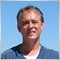9
10 797

First, a few words about testing procedure. Before starting to test, the testing subsystem loads the expert, sets its parameters previously defined by the user, and calls the init() function. Then the Tester plays through the generated sequence and calls the start() function every time. When the test sequence is exhausted, the Tester calls the deinit() function. At this, the entire trading history generated during testing is available. The expert efficiency can be analyzed at this moment.

The CalculateSummary function below provides calculation of test results, i.e., the data given in the standard report of the Strategy Tester.

```void CalculateSummary(double initial_deposit)
{
int    sequence=0, profitseqs=0, lossseqs=0;
double sequential=0.0, prevprofit=EMPTY_VALUE, drawdownpercent, drawdown;
double maxpeak=initial_deposit, minpeak=initial_deposit, balance=initial_deposit;
//---- initialize summaries
InitializeSummaries(initial_deposit);
//----
{
if(!OrderSelect(i,SELECT_BY_POS,MODE_HISTORY)) continue;
int type=OrderType();
//---- initial balance not considered
if(i==0 && type==OP_BALANCE) continue;
//---- calculate profit
double profit=OrderProfit()+OrderCommission()+OrderSwap();
balance+=profit;
//---- drawdown check
if(maxpeak<balance)
{
drawdown=maxpeak-minpeak;
if(maxpeak!=0.0)
{
drawdownpercent=drawdown/maxpeak*100.0;
if(RelDrawdownPercent<drawdownpercent)
{
RelDrawdownPercent=drawdownpercent;
RelDrawdown=drawdown;
}
}
if(MaxDrawdown<drawdown)
{
MaxDrawdown=drawdown;
if(maxpeak!=0.0) MaxDrawdownPercent=MaxDrawdown/maxpeak*100.0;
else MaxDrawdownPercent=100.0;
}
maxpeak=balance;
minpeak=balance;
}
if(minpeak>balance) minpeak=balance;
if(MaxLoss>balance) MaxLoss=balance;
//---- market orders only
SummaryProfit+=profit;
if(profit<0)
{
GrossLoss+=profit;
if(MinProfit>profit) MinProfit=profit;
//---- fortune changed
if(prevprofit!=EMPTY_VALUE && prevprofit>=0)
{
{
ConProfit1=sequential;
}
if(ConProfit2<sequential ||
{
ConProfit2=sequential;
}
profitseqs++;
AvgConWinners+=sequence;
sequence=0;
sequential=0.0;
}
}
else
{
GrossProfit+=profit;
if(MaxProfit<profit) MaxProfit=profit;
//---- fortune changed
if(prevprofit!=EMPTY_VALUE && prevprofit<0)
{
{
ConLoss1=sequential;
}
if(ConLoss2>sequential ||
{
ConLoss2=sequential;
}
lossseqs++;
AvgConLosers+=sequence;
sequence=0;
sequential=0.0;
}
}
sequence++;
sequential+=profit;
//----
prevprofit=profit;
}
//---- final drawdown check
drawdown=maxpeak-minpeak;
if(maxpeak!=0.0)
{
drawdownpercent=drawdown/maxpeak*100.0;
if(RelDrawdownPercent<drawdownpercent)
{
RelDrawdownPercent=drawdownpercent;
RelDrawdown=drawdown;
}
}
if(MaxDrawdown<drawdown)
{
MaxDrawdown=drawdown;
if(maxpeak!=0) MaxDrawdownPercent=MaxDrawdown/maxpeak*100.0;
else MaxDrawdownPercent=100.0;
}
if(prevprofit!=EMPTY_VALUE)
{
profit=prevprofit;
if(profit<0)
{
{
ConLoss1=sequential;
}
if(ConLoss2>sequential ||
{
ConLoss2=sequential;
}
lossseqs++;
AvgConLosers+=sequence;
}
else
{
{
ConProfit1=sequential;
}
if(ConProfit2<sequential ||
{
ConProfit2=sequential;
}
profitseqs++;
AvgConWinners+=sequence;
}
}
//---- collecting done
double dnum, profitkoef=0.0, losskoef=0.0, avgprofit=0.0, avgloss=0.0;
//---- average consecutive wins and losses
dnum=AvgConWinners;
if(profitseqs>0) AvgConWinners=dnum/profitseqs+0.5;
dnum=AvgConLosers;
if(lossseqs>0)   AvgConLosers=dnum/lossseqs+0.5;
//---- absolute values
if(GrossLoss<0.0) GrossLoss*=-1.0;
if(MinProfit<0.0) MinProfit*=-1.0;
if(ConLoss1<0.0)  ConLoss1*=-1.0;
if(ConLoss2<0.0)  ConLoss2*=-1.0;
//---- profit factor
if(GrossLoss>0.0) ProfitFactor=GrossProfit/GrossLoss;
//---- expected payoff
{
ExpectedPayoff=profitkoef*avgprofit-losskoef*avgloss;
}
//---- absolute drawdown
AbsoluteDrawdown=initial_deposit-MaxLoss;
}```

For calculations to be correct, the value of the initial deposit must be known. For this, in the init() function, the AccountBalance() function must be called that will give the balance value at the testing start.

```void init()
{
ExtInitialDeposit=AccountBalance();
}```

In the above CalculateSummary function, as well as in a standard report, the profit is calculated in the deposit currency. Other trade results, such as the "Largest profit trade" or the "Maximal consecutive loss" that are calculated on profit basis, are also measured in money terms. It is easy to recalculate the profit in points then.

```...
//---- market orders only
//---- calculate profit in points
profit=(OrderClosePrice()-OrderOpenPrice())/MarketInfo(OrderSymbol(),MODE_POINT);
SummaryProfit+=profit;
...```

The obtained results can be written into the report file using the WriteReport function.

```void WriteReport(string report_name)
{
int handle=FileOpen(report_name,FILE_CSV|FILE_WRITE,'\t');
if(handle<1) return;
//----
FileWrite(handle,"Initial deposit           ",InitialDeposit);
FileWrite(handle,"Total net profit          ",SummaryProfit);
FileWrite(handle,"Gross profit              ",GrossProfit);
FileWrite(handle,"Gross loss                ",GrossLoss);
if(GrossLoss>0.0)
FileWrite(handle,"Profit factor             ",ProfitFactor);
FileWrite(handle,"Expected payoff           ",ExpectedPayoff);
FileWrite(handle,"Absolute drawdown         ",AbsoluteDrawdown);
FileWrite(handle,"Maximal drawdown          ",
MaxDrawdown,
StringConcatenate("(",MaxDrawdownPercent,"%)"));
FileWrite(handle,"Relative drawdown         ",
StringConcatenate(RelDrawdownPercent,"%"),
StringConcatenate("(",RelDrawdown,")"));
FileWrite(handle,"Short positions(won %)    ",
FileWrite(handle,"Long positions(won %)     ",
FileWrite(handle,"Loss trades (% of total)  ",
FileWrite(handle,"Average consecutive wins  ",AvgConWinners);
FileWrite(handle,"Average consecutive losses",AvgConLosers);
FileWrite(handle,"Maximum consecutive wins (profit in money)",
StringConcatenate("(",ConProfit1,")"));
FileWrite(handle,"Maximum consecutive losses (loss in money)",
StringConcatenate("(",-ConLoss1,")"));
FileWrite(handle,"Maximal consecutive profit (count of wins)",
ConProfit2,
FileWrite(handle,"Maximal consecutive loss (count of losses)",
-ConLoss2,
//----
FileClose(handle);
}```

An example of how these functions are used to generate a report is given below.

```void deinit()
{
if(!IsOptimization())
{
if(!IsTesting()) ExtInitialDeposit=CalculateInitialDeposit();
CalculateSummary(ExtInitialDeposit);
WriteReport("MACD_Sample_Report.txt");
}
}```

You can see that the reports can be generated not only after testing, but at deinitialization of live expert advisor. You may ask how to get to know the initial deposit size if the account history was downloaded in the terminal only partially (for example, only one-month history was requested in the Account History tab). The CalculateInitialDeposit function helps to solve this problem.

```double CalculateInitialDeposit()
{
double initial_deposit=AccountBalance();
//----
for(int i=HistoryTotal()-1; i>=0; i--)
{
if(!OrderSelect(i,SELECT_BY_POS,MODE_HISTORY)) continue;
int type=OrderType();
//---- initial balance not considered
if(i==0 && type==OP_BALANCE) break;
{
//---- calculate profit
double profit=OrderProfit()+OrderCommission()+OrderSwap();
//---- and decrease balance
initial_deposit-=profit;
}
if(type==OP_BALANCE || type==OP_CREDIT)
initial_deposit-=OrderProfit();
}
//----
return(initial_deposit);
}```

This is the way in which the reports are generated in MetaTrader 4 Client Terminal.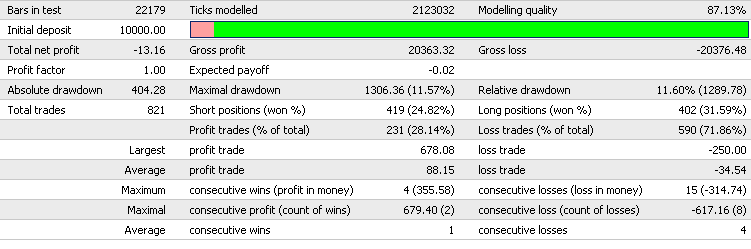It can be compared to data calculated using the exposed program.

```Initial deposit             10000
Total net profit            -13.16
Gross profit                20363.32
Gross loss                  20376.48
Profit factor               0.99935416
Expected payoff             -0.01602923
Absolute drawdown           404.28
Maximal drawdown            1306.36 (11.5677%)
Relative drawdown           11.5966%    (1289.78)
Short positions(won %)      419 (24.821%)
Long positions(won %)       402 (31.592%)
Profit trades (% of total)  231 (28.1364%)
Loss trades (% of total)    590 (71.8636%)
Average consecutive wins    1
Average consecutive losses  4
Maximum consecutive wins (profit in money)  4   (355.58)
Maximum consecutive losses (loss in money)  15  (-314.74)
Maximal consecutive profit (count of wins)  679.4   (2)
Maximal consecutive loss (count of losses)  -617.16 (8)```

```#include <SummaryReport.mq4>

double ExtInitialDeposit;```

Translated from Russian by MetaQuotes Software Corp.
Original article: /ru/articles/1403

Translated from Russian by MetaQuotes Software Corp.
Original article: https://www.mql5.com/ru/articles/1403

Attached files |
Last comments | Go to discussion (9)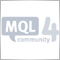| 31 Mar 2010 at 09:59
"profit in points" and "calculate profit in points" i can't forget out!
you're expert making money on backtest cool but switch to "calculate profit in points" some expert show as loosing mony ? i mean what exactly calculate profit in points mean ?

here we are again!
the normal profit calculation is
//---- calculate profit
double profit=OrderProfit()+OrderCommission()+OrderSwap();
profit in point calculation is

//---- calculate profit in points
profit=(OrderClosePrice()-OrderOpenPrice())/MarketInfo(OrderSymbol(),MODE_POINT);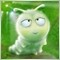| 15 Sep 2010 at 16:52

mark| 30 Jan 2011 at 02:35
Good. Then I can use Excel or MATLAB to draw a picture.| 15 Nov 2011 at 12:40
does this work for optimisation also?| 5 Sep 2012 at 04:42

Hi Slawa et al,

Very much a NuB here.

I need a Back Test and Optimization Report Summary that doesn't include all of the trades. I'm hoping to add in the picture of the graph as well. But this looks like just what I need thanks (< 8)

Before I start, what and where are the two programs that generate the Mt4 Optimization and Back Test reports? Are they compiled (C++) MQL4 and are they directly accessible by us? It would be great if they were as I just need to eliminate the large lists of all of the trades and could possibly temporarily disable this aspect of them. Or just have to separate report Summary versions: One with the trades included and one without.

But *.mqh files go into the include folder and not *.mq4 files done't they? I tried it both as an mq4 and an mqh file and in both instances the compiler checks them out as being just fine themselves. However as soon as the EA is compiled, it goes crazy as it encounters 2 init, 2 deinit sections and flags these as errors and a whole LOT of: ')' - unbalanced right parenthesis errors. I've looked it over and I can't see how it can work in the setup with putting basically what are 2 complete EAs together and combining the code into one init section and one deinit section.

So I tried 'Copying and pasting' everything from your ReportSummary program that is in the init and deinit sections into an EA and 'remarked these out in your report EA and I can get these two functioning alright this way, but then it does the same thing with the 'start' function which being the body of the program is huge. Thus it would basically melding the two EAs together. But I know that include files can and do work very well for such things. But aren't they usually just a bunch of indivdiual function definitions etc so that one can just call them from any EA at any time without having to remake them every time?

Their is little doubt that you and the other folks here know a LOT more about this than I do and that I am making a fundamental error right from the very start? I'm fairly certain that what I have been trying: combining them both into one physical EA, is not the problem and approach to use. What fundamental mistake am I making right at the very start of this please that thwarts the whole thing for me?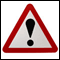Requirements Applicable to Articles Offered for Publishing at MQL4.com

Requirements Applicable to Articles Offered for Publishing at MQL4.com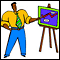Expert Advisor Sample

The principles of MQL4-programs development are shown on sample of creating a simple Expert Advisor system based on the standard MACD indicator.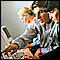Genetic Algorithms: Mathematics

Genetic (evolutionary) algorithms are used for optimization purposes. An example of such purpose can be neuronet learning, i.e., selection of such weight values that allow reaching the minimum error. At this, the genetic algorithm is based on the random search method.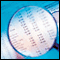One-Minute Data Modelling Quality Rating

One-Minute Data Modelling Quality Rating